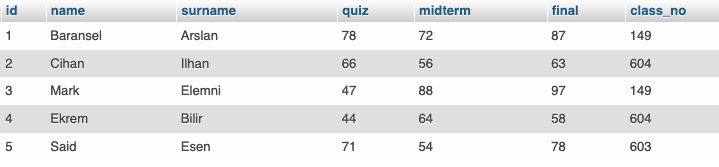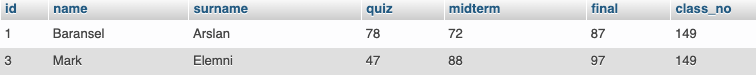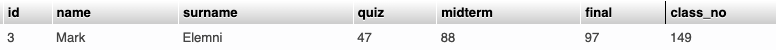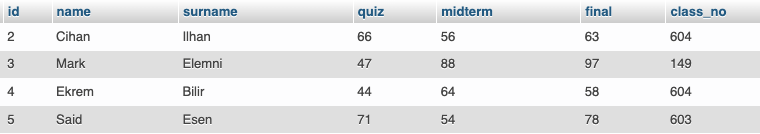`AND`, `OR`, and `NOT` Operators are logical SQL query commands used for multiple conditional situations on a table. Let’s take a look at the table named students that we created earlier.For example, the class number `149` has more than one student, let’s see right away;

``````SELECT * FROM students WHERE class_no = 149
``````As you can see, two students are in the same class.

## AND Operator

Here, we want to draw the line with both the student whose class number is `149` and the information that his final mark is more than `90`. In other words, we want to obtain rows that satisfy more than one condition, for this we use the `AND` operator.

Here, if we say `1` when the condition is met, `0` if the condition is not met, our result will be met only if both conditions are met, and in other cases, our result will be `0`, that is, it will not be met. That is, all conditions must be met for results to be achieved.

CONDITION 1 CONDITION 2 RESULT
0 0 0
0 1 0
1 0 0
1 1 1
``````SELECT * FROM students WHERE class_no = 149 AND final > 90
``````We have obtained one line satisfying these conditions.

## OR Operator

We just pulled the data that satisfies two different conditions at the same time. Now, let’s pull the data, as well as the rows that satisfy one of the two conditions, for this we use the `OR` operator for SQL queries; Here again, assuming that there are two conditions in the same way, the result is not provided in cases where the two conditions are not met only, but the result is provided in all other cases. That is, at least one condition must be met.

CONDITION 1 CONDITION 2 RESULT
0 0 0
0 1 1
1 0 1
1 1 1

Now let’s take the data on the table with the name of the class number `149` or the final grade above `90`.

``````SELECT * FROM students WHERE class_no = 149 OR final > 90
``````

## NOT Operator

It is used to retrieve all data outside the specified expression. In other words, in the opposite case, the result is provided. Let’s see our table now.

For example, let’s take all students whose name is not `Baransel`.

``````SELECT * FROM students WHERE NOT name = "Baransel"
``````

Note: Another point to note here is that we write in quotation marks for columns consisting of characters.We have obtained a table for all the students you see, except for `Baransel`.

Let’s end the post with one final example.

Let’s take the lines without the student name `Baransel` and the final grade is less than 60 or greater than 90.

``````SELECT * FROM students WHERE NOT (name = "Baransel") AND (final < 60 OR final > 90)
``````